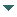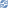# gvSIG

##### Personal tools
You are here: Home Circle
gvSIG DesktopgvSIG Desktop

Cached time 11/21/13 11:24:39# Circle

Up one level
 ◄ Previous Surface Next: Operations ►

The circles are represented on gvSIG with the Circle interface. To create a circle we can use the generic method create from the manager and, after that, establish the values of the center and radius. If we have a Point object (0,0 in this example) and we know the radius (5), we can create a circle with the following steps:

```Point centerPoint = geometryManager.createPoint(0, 0, SUBTYPES.GEOM2D);
Circle circle = (Circle)geometryManager.create(TYPES.CIRCLE, SUBTYPES.GEOM2D);
circle.setPoints(centerPoint, 5);
```

Another option is to create a circle with a center and a point in the circle's circumference diameter. The next example let us to create a circle like the created in the first example:

```Point centerPoint = geometryManager.createPoint(0, 0, SUBTYPES.GEOM2D);
Point radiousPoint = geometryManager.createPoint(0, 5, SUBTYPES.GEOM2D);
Circle circle = (Circle)geometryManager.create(TYPES.CIRCLE, SUBTYPES.GEOM2D);
```

The third option is to create a circumference with three points because there's only one which pass on them. To do that, we must create the three points and to invoke the setPoints method:

```Point point1 = geometryManager.createPoint(0, 0, SUBTYPES.GEOM2D);
Point point2 = geometryManager.createPoint(5, 5, SUBTYPES.GEOM2D);
Point point3 = geometryManager.createPoint(7, 7, SUBTYPES.GEOM2D);
Circle circle = (Circle)geometryManager.create(TYPES.CIRCLE, SUBTYPES.GEOM2D);
circle.setPoints(point1, point2, point3);
```
 ◄ Previous Surface Next: Operations ►